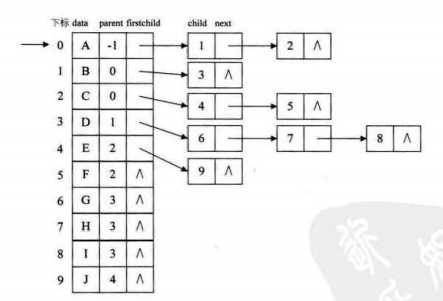# 树结构存储表示

```对于双亲表示法：我们先将双亲结点存入，我们每插入一个结点都是知道双亲结点位置的，数据可以直接插入。使用顺序存储结构更加方便

## （一）双亲表示法

```以双亲作为索引的关键词的一种存储方式

```#include<iostream>
#include<cstring>
const int MAXN=100;
using namespace std;

struct node
{//双亲表示法.
char val;//值
int par;//父亲
};
node L[MAXN];
int length;
bool vis[MAXN];
void print(int root)
{//这个程序不用担心产生环.
if(root<length)
{
cout<<L[root].val<<" "; vis[root]=true;
for(int i=0;i<length;i++)
if(!vis[i]&&L[i].par==root) print(i);　　　　　　　//这个地方是有瑕疵的,其实不必有vis的判断,因为树本身的性质决定了它不可能存在图的遍历中可能存在的环,也就排除了重复访问的可能性.
}
return ;
}

void init(int n)
{
for(int i=0;i<n;i++) L[i].par=-1,vis[i]=false;

}

int main()
{
int n;
char chx,chy;
cin>>n;
length=n;
init(n);
for(int i=0;i<n-1;i++)
{
int x,y;
cin>>x>>chx>>y>>chy;
L[x].val=chx; L[y].val=chy;
L[y].par=x;
}
print(0);
return 0;
}
/*
10
0 a 1 b
0 a 2 c
1 b 3 d
3 d 6 g
3 d 7 h
3 d 8 i
2 c 4 e
2 c 5 f
4 e 9 j
a b d g h i c e j f
*/```

int par[maxn];//par[i]表示i结点的父亲下标     int val[maxn];//val[i]表示i结点对应的值.

`二.孩子表示法.`
``` 1 #include<iostream>
2 #include<cstring>
3 using namespace std;
4
5 //孩子表示法;
6 //即每个树结点都有孩子索引域
7
8 struct node
9 {//degree=2
10     char val;
11     int left,right;
12 }a;
13 int n;
14 void init()
15 {
16     for(int i=0;i<n;i++) a[i].val=1,a[i].left=a[i].right=-1;
17 }
18
19 void print(int root)
20 {
21     if(root<n)
22     {
23         cout<<a[root].val<<" ";
24         if(a[root].left!=-1) print(a[root].left);
25         if(a[root].right!=-1) print(a[root].right);
26     }
27     return ;
28 }
29
30
31 int main()
32 {
33     int cnt=0,x,y;
34     char chx,chy;
35     cin>>n;
36     init();
37     while(1)
38     {
39         if(cnt==n-1) break;
40         cin>>x>>chx>>y>>chy;
41         a[x].val=chx; a[y].val=chy;
42         if(a[x].left==-1) a[x].left=y;
43         else a[x].right=y;
44         cnt++;
45     }
46     print(0);
47     return 0;
48 }```

```1 struct node
2 {//degree=2
3     char val;
4     int par;
5     int left,right;
6 }```

```1 struct node
2 {//degree=2
3     char val;4 　　 int par;
5     int child[max_degree];
6 }````两种线性表的结合是不是很不错呢?具体怎么运用呢?自己琢磨去!三.孩子兄弟法.下面就是孩子兄弟法了,具体是:`
```1 struct node
2 {
3     char val;
4     node *firstchild,*next_sibling;
5 };```
`可以看出,结点的内容是存储每一个结点的第一个孩子以及此结点的兄弟.可以看出,我们既优化了每个父亲对多个孩子的索引的简化,又能使得每个结点可以轻松的访问它的众多兄弟,其实在xpath和dom树中对结点的兄弟的获取就是靠着这种结构而构造成的.`

``` 1 #include<iostream>
2 #include<cstring>
3 using namespace std;
4
5 //孩子表示法;
6 //即每个树结点都有孩子索引域
7 struct node
8 {
9     char val;
10     node *firstchild,*next_sibling;
11 }a;
12 int n;
13 void init()
14 {
15     for(int i=0;i<n;i++) a[i].val=1,a[i].firstchild=a[i].next_sibling=NULL;
16 }
17
18 void print(node *root)
19 {
20     if(root!=NULL)
21     {
22         cout<<root->val<<" ";
23         node* temp=root->firstchild;
24         while(temp)
25         {
26             print(temp);
27             temp=temp->next_sibling;
28         }
29     }
30     return ;
31 }
32
33
34 int main()
35 {
36     int cnt=0,x,y;
37     char chx,chy;
38     cin>>n;
39     init();
40     while(1)
41     {
42         if(cnt==n-1) break;
43         cin>>x>>chx>>y>>chy;
44         a[x].val=chx; a[y].val=chy;
45         if(!a[x].firstchild) a[x].firstchild=&a[y];
46         else
47         {
48             node * temp=a[x].firstchild,*fa=NULL;
49             while(temp) fa=temp,temp=temp->next_sibling;
50             fa->next_sibling=&a[y];
51         }
52         cnt++;
53     }
54     print(&a);
55     return 0;
56 }```

`以下是两份我觉得写的很不错的博文.值得参考.https://www.cnblogs.com/jpfss/p/10842521.html　　梗概https://www.cnblogs.com/ssyfj/p/9459887.html　　优化.`

(0)
(0)